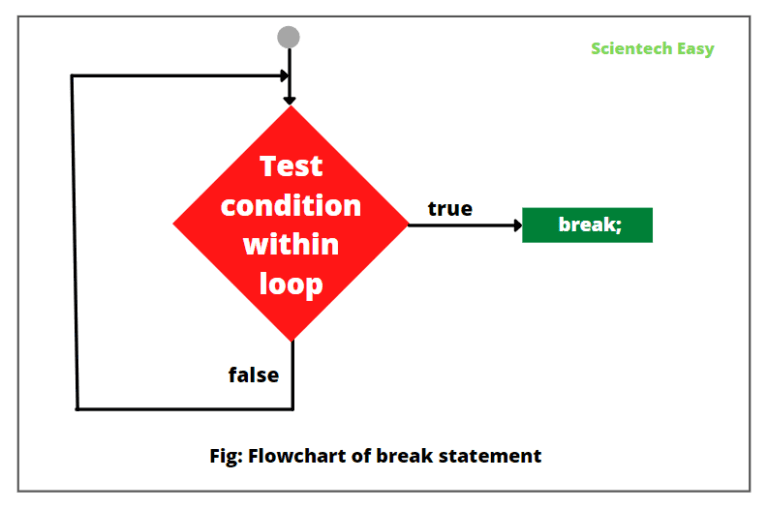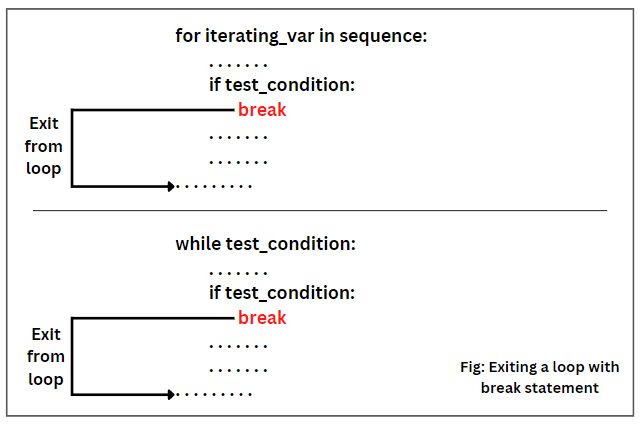# Break Statement in Python with Example

A break statement in Python is a loop control statement that ends the present loop statement and instructs Python to execute the next statement immediately following the loop.

It provides a greater control over the execution of statements in a loop.

When Python encounters a break statement inside a loop body, the loop immediately ends at a specified condition if the test condition is true.

Python then transfers the control to execute the next line of code (if any) immediately outside the loop, even if the loop condition has not become false or the sequence of elements has not been completely iterated over.

We can use the break statement in all types of loops, such as for loop, while loop, and nested loops.

## Syntax of Break Statement

The general syntax for a break statement in Python is as follows:

```# Jump statement
break;
```

The flowchart diagram of break statement in Python has shown in the below figure.From the above flowchart diagram, it is clear that if the Python evaluates the test condition within a loop to true, then the loop ends immediately and the execution control jumps to the next statement following the loop.

## Use of Break Statement in Python

There are three important uses of break statement in Python programming language that is as:

1. We can use the break statement inside the loop body to come out of it. Look at the below figure to understand clearly.2. We can also use it inside the nested loops. In nested loops, a break statement ends the innermost loop and instructs the Python to execute the statement that follows the immediately terminated block.

3. A break statement is commonly used to prevent the execution of the else statement.

## Example Program based on Break

Example 1:

Let’s take a simple program in which we will use a break statement inside the for loop for breaking the loop.

```# Use of break statement inside the for loop.
for x in range(1, 11):
if x == 5:
break # Breaking a loop.
print("X = ",x)
```
```Output:
X =  1
X =  2
X =  3
X =  4
```

In the above code, when x == 5, then the for loop automatically breaks. Therefore, the program only prints the current values from 1 to 4.

Example 2:

Let’s write a program in which we will use break statement inside while loop to exit from loop.

```# Use of break statement inside the while loop.
i = 1
while i <= 10:
if i == 5:
break # Breaking a loop.
print("I = ",i)
i = i + 1
```
```Output:
I =  1
I =  2
I =  3
I =  4
```

Example 3:

```for letter in 'Python':
if letter == 't':
break
print('Current letter is ',letter)
```
```Output:
Current letter is  P
Current letter is  y
```

Example 4:

Let’s write a program in which we will use break statement inside the inner for loop to exit from loop.

```# Use of break inside the inner for loop.
# Outer for loop.
for x in range(1,4):
for y in 0, 1, 2, 3: # Inner for loop.
if(x == 2 and y == 2):
break # Using break statement inside inner for loop.
print(x,y)
```
```Output:
1 0
1 1
1 2
1 3
2 0
2 1
3 0
3 1
3 2
3 3
```

Example 5:

Let’s write a program to use the break statement in a for loop iterating over a list. Take a number from the user that will be searched in the list. If it is found, the loop ends with the found message.

```num = int(input('Enter a number: '))
num_list = [20, 40, 60, 80, 100, 120]
for n in num_list:
if n == num:
print('Entered number found in the list.')
break
else:
```
```Output:
Enter a number: 40
Entered number found in the list.
Enter a number: 30